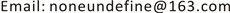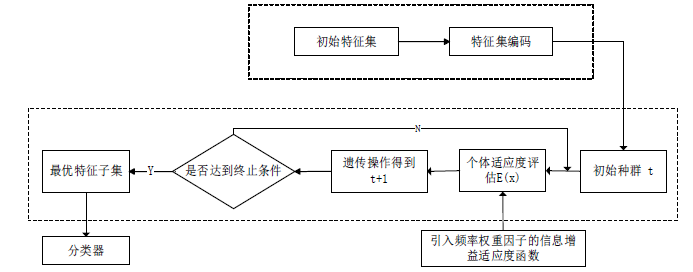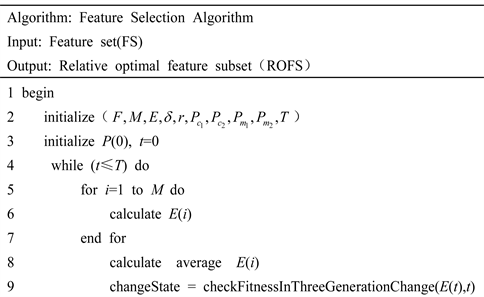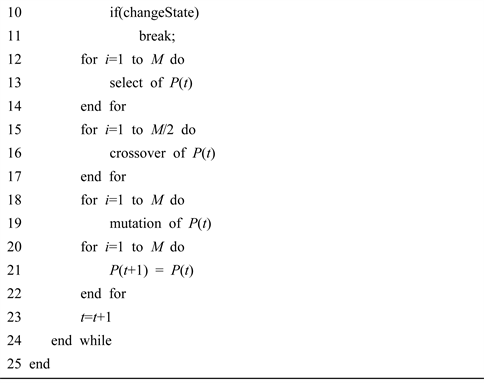﻿ 基于WIG-GA特征选择算法的恶意代码检测方法 Malicious Code Detection Method Based on WIG-GA Feature Selection Algorithm

Computer Science and Application
Vol.08 No.03(2018), Article ID:24175,9 pages
10.12677/CSA.2018.83031

Malicious Code Detection Method Based on WIG-GA Feature Selection Algorithm

Guan Wang1,2, Ranran Hao1,2, Shangwei Gao1

1College of Computer Science, Beijing University of Technology, Beijing

2Key Laboratory of Trustworthy Computing in Beijing, BeijingReceived: Mar. 5th, 2018; accepted: Mar. 19th, 2018; published: Mar. 27th, 2018ABSTRACT

In order to solve the problem of feature dimensionality in current malicious code detection, this paper proposes a method of feature selection of malicious code based on information gain algorithm which introduces frequency weight factor and genetic algorithm. This method can select the optimal feature subset that can effectively distinguish between normal code and malicious code, and achieve dimensionality reduction. This method uses the strong global search ability of genetic algorithm to search the feature subset. At the same time, the information gain algorithm based on the frequency weighting factor is used as the fitness evaluation of the feature subset. At last, we use a variety of popular classifiers to learn and verify. Experiments show that this method can effectively reduce the dimensions of features in malicious code detection and effectively improve the learning efficiency and accuracy of the classifier.

Keywords:Malicious Code, Dimensionality Reduction, Genetic Algorithm, Classification

1北京工业大学计算机学院，北京

2可信计算北京市重点实验室，北京1. 引言

2. 相关工作

3. 特征提取

N-gram模型被广泛应用在自然语言处理领域，在恶意代码特征提取方面也有很好的效果。本文采用变长的N-gram模型提取字节层特征。在恶意代码检测中，N-gram是使用一个长度为N的滑动窗口对二进制序列进行收集，得到长度为N的字节序列字符串。例如一段二进制字节序列S = (14, 8B, 3C, 01, 15, 2C)二进制字节序列，当N为3的时候，可以获得四段子序列分别为S1 = (14, 8B, 3C,), S2 = (8B, 3C, 01), S3 = (3C, 01, 15), S4 = (01, 15, 2C)。但是定长的N-gram算法容易把有意义的字节序列断开，从而破坏能够区分正常代码和恶意代码的字节序列。变长的N-gram模型可以弥补这个缺点，并且取得了不错的效果  。所以本文采用引入专家投票算法的N-gram模型进行程序字节层特征提取，并通过Trie树的数据结构进行存储，将提取的字节序列特征以及特征出现的频率保存下来，然后再通过本文提出的特征选择方法进行特征属性的降维，最后将选择出的最优特征子集向量供后续的分类器使用。

4. 基于WIG-GA特征选择方法

N-gram模型提取的字节特征较多维度较大  ，大量的高维度特征会造成分类器的分类性能降低、分类精度下降等很多问题，因此对提取的字节特征进行降维，选出能够代表分类空间的最优特征子集是非常必要的。目前常用的特征选择方法有信息增益(IG)、特征权(TS)、文档频率(DF)等，这些方法虽然能通过对特征属性的删减达到降维的目的，但是可能会使特征空间丢失掉原来特征集所包含的全部信息。最优特征子集的选择问题实质上是一个特征组合的寻优问题，遗传算法(GA)，也被称为进化算法，是一种受进化论启发，通过模拟自然进化过程搜索最优解的算法。遗传算法在组合优化问题中得到了很好的应用，也引起了很多学者的关注，文献  针对遗传算法在组合优化问题中提出了几种不同的适应度函数的构造方法。本文提出一种基于引入频率权重因子的信息增益和遗传算法相结合的特征选择方法(introduced frequency weight factor information gain with genetic algorithm, WIG-GA)，将遗传算法作为搜索策略，结合引入频率权重因子的信息增益算法作为评价函数，经过选择、交叉、变异操作，实现特征维度缩减，选取出最有效的特征子集，供分类器学习与预测。

4.1. 引入频率权重因子的信息增益算法(WIG)

$IG\left(M,Y\right)=H\left(M\right)-H\left(M|Y\right)$ (1)Figure 1. Feature selection process

$H\left(M\right)=-{\sum }_{i=1}^{c}\frac{{x}_{i}}{M}{\mathrm{log}}_{2}\frac{{x}_{i}}{M}$ (2)

$H\left(M|Y\right)=-{\sum }_{j=1}^{n}\frac{{y}_{j}}{M}{\sum }_{i=1}^{c}\frac{{y}_{ij}}{{y}_{j}}{\mathrm{log}}_{2}\frac{{y}_{ij}}{{y}_{j}}$ (3)

$IG\left(M,Y\right)=-{\sum }_{i=1}^{c}\frac{{x}_{i}}{M}{\mathrm{log}}_{2}\frac{{x}_{i}}{M}+{\sum }_{j=1}^{n}\frac{{y}_{j}}{M}{\sum }_{i=1}^{c}\frac{{y}_{ij}}{{y}_{j}}{\mathrm{log}}_{2}\frac{{y}_{ij}}{{y}_{j}}$ (4)

${\lambda }_{ci}={\sum }_{k=1}^{m}\frac{{f}_{ik}\left({Y}_{i}\right)-{\mathrm{min}}_{1\le t\le n}\left({f}_{ik}\left({Y}_{t}\right)\right)}{{\mathrm{max}}_{1\le t\le n}\left({f}_{ik}\left({Y}_{t}\right)\right)-{\mathrm{min}}_{1\le t\le n}\left({f}_{ik}\left({Y}_{t}\right)\right)}$ (5)

$\lambda ={\lambda }_{ci}/\sqrt{{\sum }_{i=1}^{n}\text{ }{\lambda }_{ci}^{2}}$ (6)

$IG\left(M,Y\right)=-{\sum }_{i=1}^{c}\frac{{x}_{i}}{M}{\mathrm{log}}_{2}\frac{{x}_{i}}{M}+\lambda \left({\sum }_{j=1}^{n}\frac{{y}_{j}}{M}{\sum }_{i=1}^{c}\frac{{y}_{ij}}{{y}_{j}}{\mathrm{log}}_{2}\frac{{y}_{ij}}{{y}_{j}}\right)$ (7)

4.2. 基于WIG-GA算法的特征选择方案

4.2.1. 个体编码与初始种群的产生

4.2.2. 适应度评估函数设计

$I{G}_{\text{avg}}=\frac{{\sum }_{i=1}^{n}\text{ }code\left({s}_{i}\right)\ast IG\left({s}_{i}\right)}{{\sum }_{i=1}^{n}\text{ }code\left({s}_{i}\right)}$ (8)

$E\left(x\right)=I{G}_{\text{avg}}-\Phi \left(x\right)$ (9)

4.2.3. 遗传操作

1) 选择算子的设计

$P=\frac{E\left({S}_{i}\right)}{{\sum }_{i=1}^{n}\text{ }E\left({S}_{i}\right)}$ (10)

2) 交叉算子和变异算子的设计

${P}_{c}=\left\{\begin{array}{l}{P}_{{c}_{1}}-\frac{\left({P}_{{c}_{1}}-{P}_{{c}_{2}}\right)\left({E}^{\prime }-{E}_{\text{avg}}\right)}{{E}_{\mathrm{max}}-{E}_{\text{avg}}},\text{\hspace{0.17em}}\text{\hspace{0.17em}}\text{\hspace{0.17em}}\text{\hspace{0.17em}}E\ge {E}_{\text{avg}}\\ {P}_{{c}_{1}},\text{\hspace{0.17em}}\text{\hspace{0.17em}}\text{\hspace{0.17em}}\text{\hspace{0.17em}}\text{\hspace{0.17em}}\text{\hspace{0.17em}}\text{\hspace{0.17em}}\text{\hspace{0.17em}}\text{\hspace{0.17em}}\text{\hspace{0.17em}}\text{\hspace{0.17em}}\text{\hspace{0.17em}}\text{\hspace{0.17em}}\text{\hspace{0.17em}}\text{\hspace{0.17em}}\text{\hspace{0.17em}}\text{\hspace{0.17em}}\text{\hspace{0.17em}}\text{\hspace{0.17em}}\text{\hspace{0.17em}}\text{\hspace{0.17em}}\text{\hspace{0.17em}}\text{\hspace{0.17em}}\text{\hspace{0.17em}}\text{\hspace{0.17em}}\text{\hspace{0.17em}}\text{\hspace{0.17em}}\text{\hspace{0.17em}}\text{\hspace{0.17em}}\text{\hspace{0.17em}}\text{\hspace{0.17em}}\text{\hspace{0.17em}}\text{\hspace{0.17em}}\text{\hspace{0.17em}}\text{\hspace{0.17em}}\text{\hspace{0.17em}}\text{ }\text{ }{E}^{\prime }<{E}_{\text{avg}}\end{array}$ (11)

${P}_{m}=\left\{\begin{array}{l}{P}_{{m}_{1}}-\frac{\left({P}_{{m}_{1}}-{P}_{{m}_{2}}\right)\left({E}_{\mathrm{max}}-E\right)}{{E}_{\mathrm{max}}-{E}_{\text{avg}}},\text{\hspace{0.17em}}\text{\hspace{0.17em}}\text{\hspace{0.17em}}E\ge {E}_{avg}\\ {P}_{{m}_{1}},\text{\hspace{0.17em}}\text{\hspace{0.17em}}\text{\hspace{0.17em}}\text{\hspace{0.17em}}\text{\hspace{0.17em}}\text{\hspace{0.17em}}\text{\hspace{0.17em}}\text{\hspace{0.17em}}\text{\hspace{0.17em}}\text{\hspace{0.17em}}\text{\hspace{0.17em}}\text{\hspace{0.17em}}\text{\hspace{0.17em}}\text{\hspace{0.17em}}\text{\hspace{0.17em}}\text{\hspace{0.17em}}\text{\hspace{0.17em}}\text{\hspace{0.17em}}\text{\hspace{0.17em}}\text{\hspace{0.17em}}\text{\hspace{0.17em}}\text{\hspace{0.17em}}\text{\hspace{0.17em}}\text{\hspace{0.17em}}\text{\hspace{0.17em}}\text{\hspace{0.17em}}\text{\hspace{0.17em}}\text{\hspace{0.17em}}\text{\hspace{0.17em}}\text{\hspace{0.17em}}\text{\hspace{0.17em}}\text{\hspace{0.17em}}\text{ }\text{\hspace{0.17em}}\text{\hspace{0.17em}}\text{\hspace{0.17em}}\text{\hspace{0.17em}}\text{\hspace{0.17em}}E<{E}_{avg}\end{array}$ (12)

4.2.4. 算法描述5. 实验与分析

$\text{TPR}=\frac{\text{TP}}{\text{TP}+\text{FN}}$ (13)

$\text{FPR}=\frac{\text{FP}}{\text{FP}+\text{TN}}$ (14)

$\text{Accuracy}=\frac{\text{TP}+\text{TN}}{\text{TP}+\text{FN}+\text{FP}+\text{FN}}$ (15)Table 2. Classifier detection performance comparison

Random tree的准确率和检测率相对于其他分类器相对较高。综合分析，本文提出的特征选择方法可以有效的实现特征维度的减少，相对IG方法有不论是在准确率还是检测率方面都有一定的提高，并且在Native Bayes和Random tree分类算法取得了很好的检测效果。

6. 结束语

 Lee, T., Kim, D., Jeong, H., et al. (2014) Risk Prediction of Malicious Code-Infected Websites by Mining Vulnerability Features. International Journal of Security & Its Applications, 8, 291-294. https://doi.org/10.14257/ijsia.2014.8.1.27

 Abouassaleh, T., Cercone, N., Kešelj, V., et al. (2004) N-Gram-Based Detection of New Malicious Code. Proceedings of the 28th Annual International Computer Software and Applications Conference, 2, 41-42.

 Berrar, D. and Dubitzky, W. (2013) Information Gain. Springer, New York, 1022-1023. https://doi.org/10.1007/978-1-4419-9863-7_719

 郭宁, 孙晓妍, 林和, 等. 基于属性序约简的恶意代码检测[J]. 计算机应用, 2011, 31(4): 1006-1009.

 张福勇, 赵铁柱. 基于肯定选择分类算法的恶意代码检测方法[J]. 沈阳工业大学学报, 2016, 38(2): 206-210.

 朱红萍, 巩青歌, 雷战波. 基于遗传算法的入侵检测特征选择[J]. 计算机应用研究, 2012, 29(4): 1417-1419.

 Reddy, D.K.S., Dash, S.K. and Pujari, A.K. (2006) New Malicious Code Detection Using Variable Length n-Grams. Information Systems Security, 4332, 307-323.

 李盟, 贾晓启, 王蕊, 等. 一种恶意代码特征选取和建模方法[J]. 计算机应用与软件, 2015(8): 266-271.

 陈果, 邓堰. 遗传算法特征选取中的几种适应度函数构造新方法及其应用[J]. 机械科学与技术, 2011, 30(1): 124-128.